### 吉利汽车 吉利GC7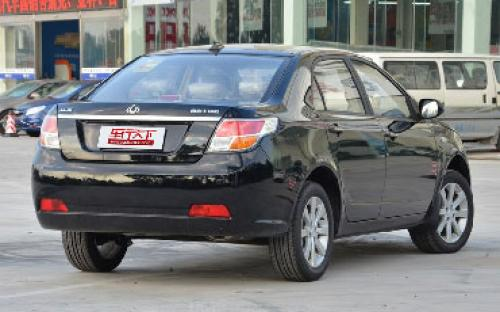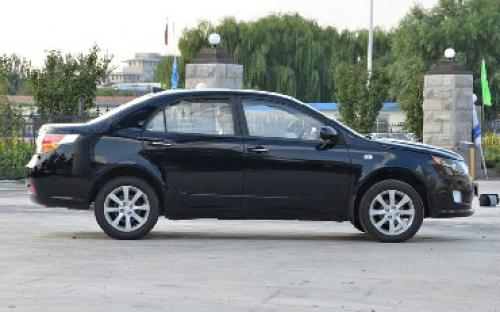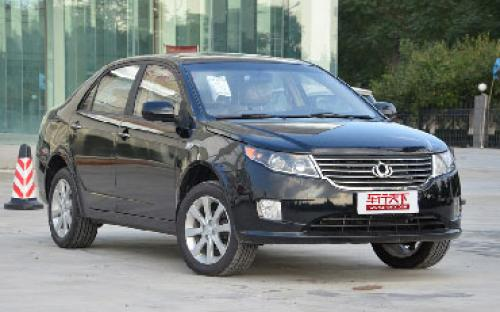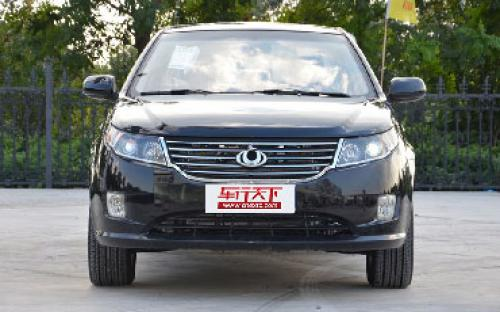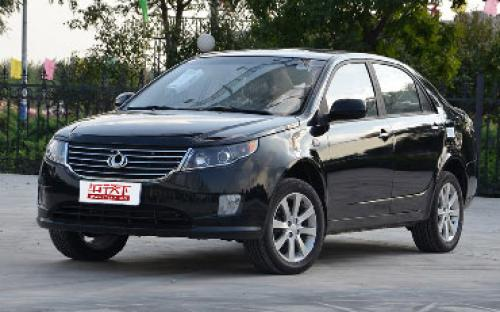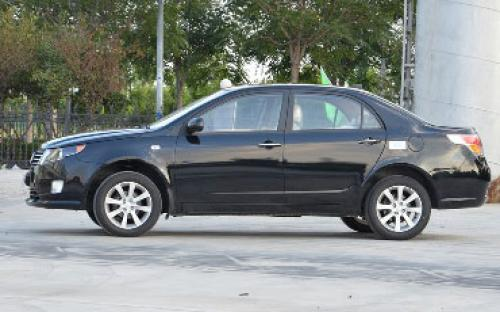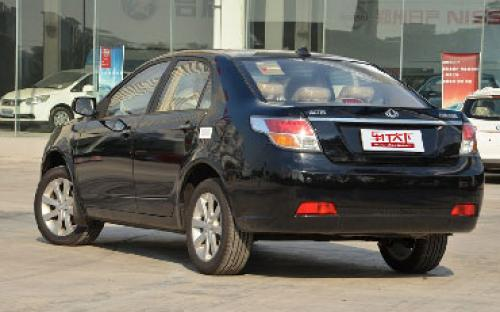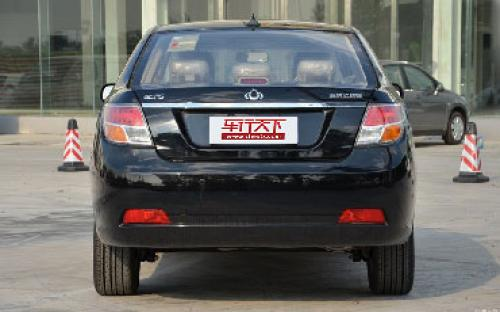5 种颜色可选2013款最低售价：6.29 万元起

4547(mm)1734(mm)1470(mm)##### 配置亮点：
• 胎压监测装置

• ISOFIX儿童座椅接口

• 车身稳定控制(ESC/ESP/DSC等)

• 电动天窗

• 定速巡航

• 后倒车雷达

• 真皮座椅

• GPS导航系统

• 氙气大灯

• 后视镜加热

• 提交

吉利汽车 吉利GC7 绕车实拍• 吉利汽车 吉利GC7 在售车型

排量 车型 厂商指导价 本地最低报价 购车工具
1.5L
6.29万
5.29万
1.00万

6.59万
5.59万
1.00万

1.8L 自动尊贵型 6挡手自一体
7.99万
6.99万
1.00万

1.8L
6.29万
5.29万
1.00万

6.59万
5.59万
1.00万

1.8L 自动尊贵型 6挡手自一体
7.99万
6.99万
1.00万

吉利汽车 吉利GC7 经销商

查看更多 >>

### 吉利汽车 吉利GC7 动力加速

吉利GC7 0-100公里加速时间分布在 0.0-秒 属于 超跑级

动力级别 加速时间 车型

吉利汽车 吉利GC7 视频

吉利汽车 吉利GC7 新闻资讯

# 吉利全球鹰GC7到店实拍解析 帅气小伙儿

车系文章 超过3722次关注

谈到自主品牌，可能大多数人的印象就是便宜，对于自主车型的外观可能并没有太高的评价。不过我们并不能因此就以点盖面，进行全盘否定，因为总有那么几款自主车型会...

# 全球鹰GC7现车销售 最高现金优惠3000元

车系文章 超过3222次关注

全球鹰GC7的外观大气稳重，不乏时尚感，受到不少网友好评；内饰在做工上面有所提升，各面板接缝均匀，按键手感都还不错，而且配置比较丰富，座椅包裹性较好；该车型...

# 上海车展发布 新款吉利全球鹰GC7谍照

车系文章 超过3384次关注

日前，一组新款吉利全球鹰GC7的谍照被曝光了出来，据悉，该车有望在今年4月份的上海国际车展上正式发布。

# 家用车的后起新秀 全球鹰GC7实拍详解

车系文章 超过5073次关注

帝豪、全球鹰、英伦是吉利汽车细分出来的三大子品牌，从目前的品牌网络来看，凭借着EC7强势开局的帝豪品牌有稳步上升的态势，而全球鹰旗下的GC2和GX2就表现平平，随...

# 一次全新的突破 吉利全球鹰GC7长沙试驾

车系文章 超过6258次关注

GC7——一款出乎所有人的意料，并且承载吉利历史使命的重要车型，它的出现甚至对于整个自主品牌来讲，都将产生深远的影响。伴随着9月8日，纳入全球鹰品牌的改款远景...

# 将年内上市 全球鹰GC7 1.3T新车信息

车系文章 超过3456次关注

全球鹰GC71.3T车型最大的亮点是动力系统，新车搭载了1.3T发动机，其最大功率为133马力，与发动机匹配的将是5速手动或6速手自一体变速箱。外观方面，全球鹰GC71.3T车...

猜你喜欢

﻿
• 快速找车
• 选择品牌
• 选择品牌
• A  奥迪
• A  阿斯顿·马丁
• A  阿尔法·罗密欧
• B  宝沃
• B  布加迪
• B  巴博斯
• B  保时捷
• B  宾利
• B  奔驰
• B  宝马
• B  本田
• B  别克
• B  标致
• B  比亚迪
• B  宝骏
• B  北汽制造
• B  北汽新能源
• B  北汽幻速
• B  北汽威旺
• B  北京汽车
• B  奔腾
• B  北汽绅宝
• C  长安
• C  长安商用
• C  长城
• C  昌河
• D  大众
• D  道奇
• D  DS
• D  东南
• D  东风风神
• D  东风风行
• D  东风小康
• D  东风风度
• D  东风
• F  福特
• F  丰田
• F  菲亚特
• F  法拉利
• F  福田
• F  福迪
• F  福汽启腾
• G  观致
• G  广汽传祺
• G  广汽吉奥
• G  GMC
• H  红旗
• H  汉腾汽车
• H  哈弗
• H  哈飞
• H  海格
• H  海马
• H  华颂
• H  黄海
• H  华泰
• H  恒天
• J  吉利汽车
• J  捷豹
• J  Jeep
• J  江淮
• J  江铃
• J  金杯
• J  九龙
• J  金旅
• K  凯翼
• K  凯迪拉克
• K  克莱斯勒
• K  科尼塞克
• K  卡威
• K  开瑞
• L  路虎
• L  林肯
• L  劳斯莱斯
• L  兰博基尼
• L  雷克萨斯
• L  铃木
• L  雷诺
• L  理念
• L  力帆
• L  莲花汽车
• L  猎豹
• L  路特斯
• L  陆风
• M  马自达
• M  MG
• M  MINI
• M  玛莎拉蒂
• M  摩根
• M  迈凯轮
• N  纳智捷
• O  欧宝
• O  讴歌
• O  欧朗
• Q  奇瑞
• Q  起亚
• Q  启辰
• R  日产
• R  荣威
• R  瑞麒
• S  三菱
• S  斯威汽车
• S  萨博
• S  smart
• S  斯柯达
• S  斯巴鲁
• S  思铭
• S  双龙
• S  上汽大通
• S  双环
• T  特斯拉
• T  腾势
• W  沃尔沃
• W  五菱汽车
• W  五十铃
• W  威兹曼
• W  威麟
• X  现代
• X  雪佛兰
• X  雪铁龙
• X  西雅特
• Y  一汽
• Y  英菲尼迪
• Y  英致
• Y  依维柯
• Y  野马汽车
• Y  永源
• Z  众泰
• Z  中华
• Z  中兴
• Z  知豆
• 选择车系
• 选择车系
• 车型对比
• 选择品牌
• 选择品牌
• A  奥迪
• A  阿斯顿·马丁
• A  阿尔法·罗密欧
• B  宝沃
• B  布加迪
• B  巴博斯
• B  保时捷
• B  宾利
• B  奔驰
• B  宝马
• B  本田
• B  别克
• B  标致
• B  比亚迪
• B  宝骏
• B  北汽制造
• B  北汽新能源
• B  北汽幻速
• B  北汽威旺
• B  北京汽车
• B  奔腾
• B  北汽绅宝
• C  长安
• C  长安商用
• C  长城
• C  昌河
• D  大众
• D  道奇
• D  DS
• D  东南
• D  东风风神
• D  东风风行
• D  东风小康
• D  东风风度
• D  东风
• F  福特
• F  丰田
• F  菲亚特
• F  法拉利
• F  福田
• F  福迪
• F  福汽启腾
• G  观致
• G  广汽传祺
• G  广汽吉奥
• G  GMC
• H  红旗
• H  汉腾汽车
• H  哈弗
• H  哈飞
• H  海格
• H  海马
• H  华颂
• H  黄海
• H  华泰
• H  恒天
• J  吉利汽车
• J  捷豹
• J  Jeep
• J  江淮
• J  江铃
• J  金杯
• J  九龙
• J  金旅
• K  凯翼
• K  凯迪拉克
• K  克莱斯勒
• K  科尼塞克
• K  卡威
• K  开瑞
• L  路虎
• L  林肯
• L  劳斯莱斯
• L  兰博基尼
• L  雷克萨斯
• L  铃木
• L  雷诺
• L  理念
• L  力帆
• L  莲花汽车
• L  猎豹
• L  路特斯
• L  陆风
• M  马自达
• M  MG
• M  MINI
• M  玛莎拉蒂
• M  摩根
• M  迈凯轮
• N  纳智捷
• O  欧宝
• O  讴歌
• O  欧朗
• Q  奇瑞
• Q  起亚
• Q  启辰
• R  日产
• R  荣威
• R  瑞麒
• S  三菱
• S  斯威汽车
• S  萨博
• S  smart
• S  斯柯达
• S  斯巴鲁
• S  思铭
• S  双龙
• S  上汽大通
• S  双环
• T  特斯拉
• T  腾势
• W  沃尔沃
• W  五菱汽车
• W  五十铃
• W  威兹曼
• W  威麟
• X  现代
• X  雪佛兰
• X  雪铁龙
• X  西雅特
• Y  一汽
• Y  英菲尼迪
• Y  英致
• Y  依维柯
• Y  野马汽车
• Y  永源
• Z  众泰
• Z  中华
• Z  中兴
• Z  知豆
• 选择车系
• 选择车系
• 选择车型
• 选择车型
• 意见反馈